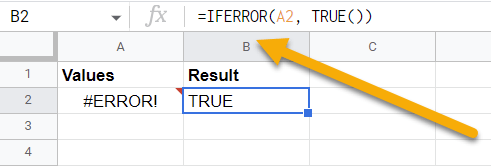# How to☝️ Use TRUE Function in Google Sheets

To use the Google Sheets TRUE function, select a blank cell, go to the Formula bar, type the formula =TRUE(), and press Enter

Take a look at the article below to learn more about the features of the TRUE function and how you can use it effectively!

## How to Use the TRUE Function in Google Sheets

Here is a step-by-step guide to help you through the process of using the TRUE function on your worksheet.

Difficulty: Beginner

Time Estimate: 5 seconds

1. Click on a blank cell where you want to apply the function.
2. Move to the Formula bar and enter the formula =TRUE().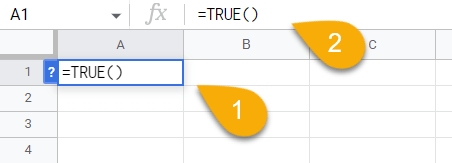3. Hit the Enter key on your keyboard.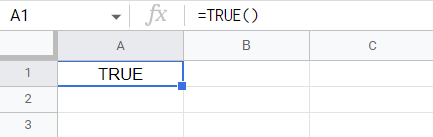Easy as ABC!

Now that you know how to add this function to your spreadsheet, let’s take a look at the uses of this tool and how you can modify it to fit your needs.

## Drilling Down into the TRUE Function

Now let’s see how this function works in detail!

### Description

The TRUE function is a logical function that returns the value TRUE.

### Purpose

If you want to test a certain condition in your spreadsheet, you might use the TRUE function in conjunction with another function. For example, you could use the TRUE function with an IF statement to check if a certain cell contains the word TRUE.

=TRUE()

### Arguments

This function does not take any arguments.

### Return Value

In this case, the TRUE value will be returned as the result.

### Usage Notes

The TRUE function can be useful if you use it in combination with other functions. Use the IF, AND, OR, or NOT functions to get the desired results.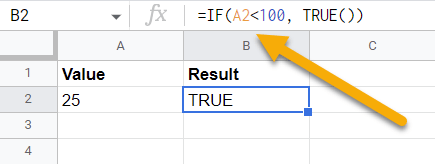Instead of the =TRUE() formula, you can enter the TRUE word directly into the cell, and the result will be the same.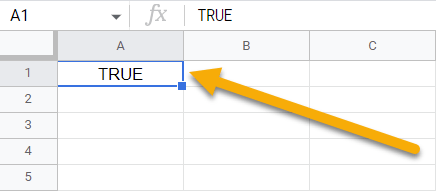### Examples

Here we are going to look at how you can combine the TRUE function with others in order to find the TRUE value easily:

1. Use the IF with the TRUE function.

=IF(A2<100, TRUE()) // Output: TRUE1. You can also apply the OR function, which will perform the calculation for you and will show you the result.

=IF(OR(A2<100, A3<100, A4<100, A5<100), TRUE()) // Output: TRUE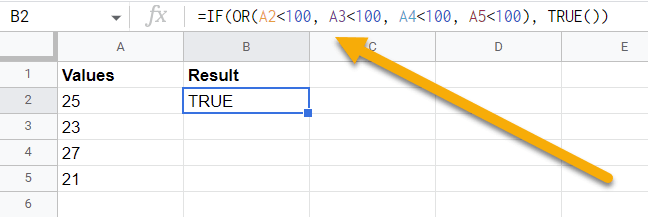1. The third function you can use is the AND function.

=IF(AND(A2<100, A3<100, A4<100, A5<100), TRUE()) // Output: TRUE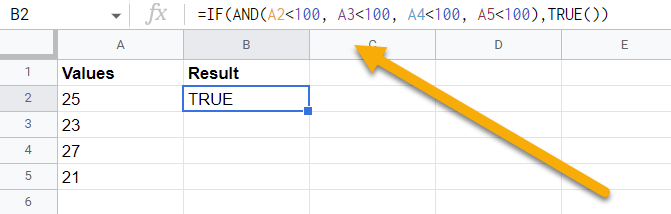1. You can also get the TRUE result using the following NOT function.

=NOT(OR(A2<20)) // Output: TRUE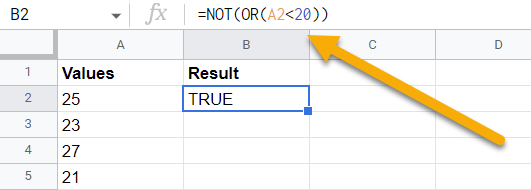1. The next function you can use to get the TRUE value is the IFERROR function.

=IFERROR(A2, TRUE()) // Output: TRUE